LIMIT OF FUNCTION

In mathematics, the limit of a function is a fundamental concept in calculus and analysis concerning the behavior of that function near a particular input.

Informally, a function f assigns an output f(x) to every input x. The function has a limit L at an input p if f(x) is “close” to L whenever x is “close” to p. In other words, f(x) becomes closer and closer to L as x moves closer and closer to p. More specifically, when f is applied to each input sufficiently close to p, the result is an output value that is arbitrarily close to L. If the inputs “close” to p are taken to values that are very different, the limit is said to not exist.

Formal definitions, first devised in the early 19th century, are given below.

History

The modern notation of placing the arrow below the limit symbol is due to Hardy in his book A Course of Pure Mathematics in 1908 (Miller 2004).

Motivation

Imagine a person walking over a landscape represented by the graph of y = f(x). His horizontal position is measured by the value of x, much like the position given by a map of the land or by a global positioning system. His altitude is given by the coordinate y. He is walking towards the horizontal position given by x = p. As he does so, he notices that his altitude approaches L. If later asked to guess the altitude over x = p, he would then answer L, even if he had never actually reached that position.

What, then, does it mean to say that his altitude approaches L? It means that his altitude gets nearer and nearer to L except for a possible small error in accuracy. For example, suppose a particular accuracy goal is set for our traveler: he must get within ten meters of L. He reports back that indeed he can get within ten meters of L, since he notes that when he is within fifty horizontal meters of p, his altitude is always ten meters or less from L.

The accuracy goal is then changed: can he get within one meter? Yes. If he is within seven horizontal meters of p, then his altitude remains within one meter of the target L. In summary, to say that the traveler’s altitude approaches L as his horizontal position approaches p means that for every target accuracy goal, there is some neighborhood of p whose altitude remains within that accuracy goal.

The initial informal statement can now be explicated:

The limit of a function f(x) as x approaches p is a number L with the following property: given any target distance from L, there is a distance from p within which the values of f(x) remain within the target distance.

This explicit statement is quite close to the formal definition of the limit of a function with values in a topological space.

Definitions

To say thatmeans that ƒ(x) can be made as close as desired to L by making x close enough, but not equal, to p.

The following definitions (known as (ε, δ)-definitions) are the generally accepted ones for the limit of a function in various contexts.

Functions on the real line

Suppose f : RR is defined on the real line and p,LR. It is said the limit of f as x approaches p is L and writtenif and only if for every real ε > 0 there exists a real δ > 0 such that for all real x, 0 < | x − p | < δ implies | f(x) − L | < ε. Note that the value of the limit does not depend on the value of f(p).

The definition can be represented symbolically as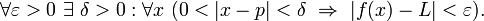0\ \exists \ \delta > 0 : \forall x\ (0 < |x – p| < \delta \ \Rightarrow \ |f(x) – L|

A more general definition applies for functions defined on subsets of the real line. Let (ab) be an open interval in R, and p a point of (ab). Let f be a real-valued function defined on at least all of (ab) \ {p}. It is then said that the limit of f as x approaches p is L, if and only if for every real ε > 0 there exists a real δ > 0 such that 0 < | x − p | < δ and x ∈ (ab) implies | f(x) − L | < ε. Note that the limit does not depend on f(p) being well-defined.

The letters ε and δ can be understood as “error” and “distance”, and in fact Cauchy used ε as an abbreviation for “error” in some of his work.”) (Grabiner 1983) In these terms, the error (ε) in the measurement of the value at the limit can be made as small as desired by reducing the distance (δ) to the limit point. As discussed below this definition also works for functions in a more general context. The idea that, δ and ε represent distances helps suggest these generalizations.

One-sided limits

Main article: One-sided limitThe limit as: x → x0+ ≠ x → x0. Therefore, the limit as x → x0 does not exist.

Alternatively x may approach p from above (right) or below (left), in which case the limits may be written as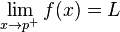orrespectively. If both of these limits are equal to L then this can be referred to as the limit of f(x) at p. Conversely, if they are not both equal to L then the limit, as such, does not exist.

A formal definition is as follows. The limit of f(x) as x approaches p from above is L if, for every ε > 0, there exists a δ > 0 such that |f(x) − L| < ε whenever 0 < x − p < δ. The limit of f(x) as x approaches p from below is L if, for every ε > 0, there exists a δ > 0 such that |f(x) − L| < ε whenever 0 < p − x < δ.

If the limit does not exist there is a non-zero oscillation.

Functions on metric spaces

Suppose M and N are subsets of metric spaces A and B, respectively, and f : MN is defined between M and N, with x ∈ M, p a limit point of M and LN. It is said that the limit of f as x approaches p is L and write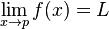if and only if for every ε > 0 there exists a δ > 0 such that, dB(f(x), L) < ε whenever 0 < dA(xp) < δ. Again, note that p need not be in the domain of f, nor does L need to be in the range of f, and even if f(p) is defined it need not be equal to L.

An alternative definition using the concept of neighbourhood is as follows:if and only if for every neighbourhood V of L in B there exists a neighbourhood U of p in A, such that f(U∩M – {p}) ⊆ V.

Functions on topological spaces

Suppose X,Y are topological spaces with Y a Hausdorff space. Let p be a limit point of Ω⊆X, and LY. For a function f : Ω → Y, it is said that the limit of f as x approaches p is L (i.e., f(x)L as xp) and writeif and only if for every open neighborhood V of L, there exists an open neighborhood U of p such that f(U∩Ω- {p}) ⊆ V. This last part of the definition can also be phrased “there exists an open punctured neighbourhood U of p such that f(U∩Ω) ⊆ V “.

Note that the domain of f does not need to contain p. If it does, then the value of f at p is irrelevant to the definition of the limit. In particular, if the domain of f is X – {p} (or all of X), then the limit of f as xp exists and is equal to L if and only if for all subsets Ω of X with limit point p the limit of the restriction of f to Ω exists and is equal to L. Sometimes this criterion is used to establish the non-existence of the two-sided limit of a function on R by showing that the one-sided limits either fail to exist or do not agree. Such a view is fundamental in the field of general topology, where limits and continuity at a point are defined in terms of special families of subsets, called filters, or generalized sequences known as nets.

Alternatively, the requirement that Y be a Hausdorff space can be relaxed to the assumption that Y be a general topological space, but then the limit of a function will not be unique. In particular, one can no longer talk about the limit of a function at a point, but rather a limit or the set of limits at a point.

A function is continuous in a limit point p of and in its domain if and only f(p) is the (or, in the general case, a) limit of f(x) as x tends to p.

Limits involving infinityImage via WikipediaThe limit of this function at infinity exists.

If the extended real line R is considered, i.e., R ∪ {-∞, ∞}, then it is possible to define limits of a function at infinity.

If f(x) is a real function, then the limit of f as x approaches infinity is L, denoted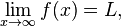if and only if for all0″ /> there exists S > 0 such that <img src=”http://upload.wikimedia.org/math/2/c/4/2c412a6f49514db4dbd890537413bcc6.png&#8221; alt=”|f(x) – L| whenever x > S.

Similarly, the limit of f as x approaches negative infinity is L, denoted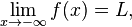if and only if for all0″ /> there exists S < 0 such that <img src=”http://upload.wikimedia.org/math/2/c/4/2c412a6f49514db4dbd890537413bcc6.png&#8221; alt=”|f(x) – L| whenever x < S.

For exampleLimits can also have infinite values, for example the limit of f as x approaches a is infinity, denotedif and only if for all0″ /> there exists δ > 0 such that\varepsilon” /> whenever | xa | < δ.

These ideas can be combined in an obvious way to produce definitions for different combinations, such as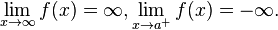For example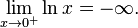Limits involving infinity are connected with the concept of asymptotes.

These notions of a limit attempt to provide a metric space interpretation to limits at infinity. However, note that these notions of a limit are consistent with the topological space definition of limit if

• a neighborhood of −∞ is defined to contain an interval [−∞, c) for some c ∈ R
• a neighborhood of ∞ is defined to contain an interval (c, ∞] where c ∈ R
• a neighborhood of aR is defined in the normal way metric space R

In this case, R is a topological space and any function of the form fX → Y with XY⊆ R is subject to the topological definition of a limit. Note that with this topological definition, it is easy to define infinite limits at finite points, which have not been defined above in the metric sense.

Alternate notation

Many authors allow for the real projective line to be used as a way to include infinite values as well as extended real line. With this notation, the extended real line is given as R ∪ {-∞, +∞} and the projective real line is R ∪ {∞} where a neighborhood of ∞ is a set of the form {x: |x|>c}. In this notation, for example,Evaluating limits at infinity for rational functionsImage via WikipediaHorizontal Asymptote about y= 4

There are three basic rules for evaluating limits at infinity for a rational function f(x) = p(x)/q(x):

• If the degree of p is greater than the degree of q, then the limit is positive or negative infinity depending on the signs of the leading coefficients;
• If the degree of p and q are equal, the limit is the leading coefficient of p divided by the leading coefficient of q;
• If the degree of p is less than the degree of q, the limit is 0.

If the limit at infinity exists, it represents a horizontal asymptote at y = L. Polynomials do not have horizontal asymptotes; they may occur with rational functions.

Complex-valued functions

The complex plane with metric d(x,y): = | xy | is also a metric space. There are two different types of limits when the complex-valued functions are considered.

Limit of a function at a point

If f is a complex-valued function, thenif and only if for all ε > 0 there exists a δ > 0 such that for all real numbers x with 0 < | xp | < δ, then <img src=”http://upload.wikimedia.org/math/2/c/4/2c412a6f49514db4dbd890537413bcc6.png&#8221; alt=”|f(x) – L| .

It is just a particular case of functions over metric spaces with both M and N are the complex plane.

Limit of a function of more than one variable

By noting that |xp| represents a distance, the definition of a limit can be extended to functions of more than one variable. In the case of a function f : R2R,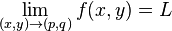if and only if

for every ε > 0 there exists a δ > 0 such that for all (x,y) with 0 < ||(x,y)(p,q)|| < δ, then |f(x,y)-L| < ε

where ||(x,y)(p,q)|| represents the Euclidean distance. This can be extended to any number of variables.

Sequential limits

Let f : XY be a mapping from a topological space X into a Hausdorff space Y, pX and LY.

The sequential limit of f as xp is L if and only if, for every sequence (xn) in X which converges to p, the sequence f(xn) converges to L.

If L is the limit (in the sense above) of f as x approaches p, then it is a sequential limit as well, however the converse need not hold in general. If in addition Y is metrizable, then L is the sequential limit of f as x approaches p if and only if it is the limit (in the sense above) of f as x approaches p.

Relationship to Continuity

The notion of the limit of a function is very closely related to the concept of continuity. A function ƒ is said to be continuous at c if it is both defined at c and its value at c equals the limit of f as x approaches c: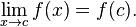If the condition 0 < |x − c| is left out of the definition of limit, then the resulting definition would be equivalent to requiring f to be continuous at c.

Properties

If a function f is real-valued, then the limit of f at p is L if and only if both the right-handed limit and left-handed limit of f at p exist and are equal to L.

The function f is continuous at p if and only if the limit of f(x) as x approaches p exists and is equal to f(p). If f : MN is a function between metric spaces M and N, then it is equivalent that f transforms every sequence in M which converges towards p into a sequence in N which converges towards f(p).

If N is a normed vector space, then the limit operation is linear in the following sense: if the limit of f(x) as x approaches p is L and the limit of g(x) as x approaches p is P, then the limit of f(x) + g(x) as x approaches p is L + P. If a is a scalar from the base field, then the limit of af(x) as x approaches p is aL.

If f is a real-valued (or complex-valued) function, then taking the limit is compatible with the algebraic operations, provided the limits on the right sides of the equations below exist (the last identity only holds if the denominator is non-zero). This fact is often called the algebraic limit theorem.In each case above, when the limits on the right do not exist, or, in the last case, when the limits in both the numerator and the denominator are zero, nonetheless the limit on the left, called an indeterminate form, may still exist—this depends on the functions f and g. These rules are also valid for one-sided limits, for the case p = ±∞, and also for infinite limits using the rules

• q + ∞ = ∞ for q ≠ −∞
• q × ∞ = ∞ if q > 0
• q × ∞ = −∞ if q < 0
• q / ∞ = 0 if q ≠ ± ∞

(see extended real number line).

Note that there is no general rule for the case q / 0; it all depends on the way 0 is approached. Indeterminate forms—for instance, 0/0, 0×∞, ∞−∞, and ∞/∞—are also not covered by these rules, but the corresponding limits can often be determined with L’Hôpital’s rule or the Squeeze theorem.

Chain rule

In general, the statement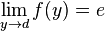, and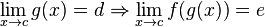,

is not true. However, this “chain rule” does hold if, in addition, either f(d) = e (i. e. f is continuous at d) or g does not take the value d near c (i. e. there exists a δ > 0 such that if 0 < | xc | < δ then | g(x) − d | > 0).

Limits of extra interest

•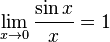•The first limit can be proven with the squeeze theorem. For 0 < x < π/2:

sinx < x < tanx.

Dividing everything by sin(x) yields

<img src=”http://upload.wikimedia.org/math/f/9/3/f93c35797c17d9706ee8db26770679d5.png&#8221; alt=”1 < \frac{x}{\sin x}
<img src=”http://upload.wikimedia.org/math/f/9/6/f96fbcc4c95200d900f5e21b9f478c9d.png&#8221; alt=”1 < \frac{x}{\sin x}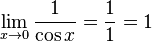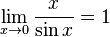L’Hôpital’s rule

This rule uses derivatives and has a conditional usage. (It can only be directly used on limits that “equal” 0/0 or ±∞/±∞. Other indeterminate forms require some algebraic manipulation usually involving setting the limit equal to y, taking the natural logarithm of both sides, and then applying l’Hôpital’s rule.)

•For example: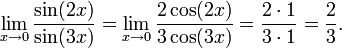Summations and integrals

A short way to write the limitis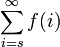.

A short way to write the limitis.

A short way to write the limitis.

RELATED POST ::Welcome to my blog. My name is Nico. Admin of this blog. I am a student majoring in mathematics who dreams of becoming a professor of mathematics. I live in Kwadungan, Ngawi, East Java. Hopefully in all the posts I can make a good learning material to the intellectual life of the nation. After the read, leave a comment. I always accept criticism suggestion to build a better me again .. Thanks for visiting .. : mrgreen:

Posted on July 23, 2011, in education and tagged , , , , , , , . Bookmark the permalink. 7 Comments.

1.snappages.com

Great site. Your blogs are very fascinating and I will tell my friends.

2.http://Www.Tarsusataturklisesi.com/

Many thanks for your really good information. They’re so valuable.

3.Johnson Bank

I am so impressed I had to save it so I continously go back and read things I may have skimmed

4.acai berry dietary supplement

Here a ton of information here. Thanks! I’ll be back for more

5.NICO VENGEANCE

usul yang merarik… baiklah akan saya laksanakan mulai bulan depan.. soalnya saya udah jadwal posting sampai akhir bulan ini.

6.Miftahgeek

Punten kang, gimana kalo pembahasan berikutnya cukup satu topik per satu topik, namun lebih mendalam, termasuk dengan contoh2 soal yang terkait 🙂